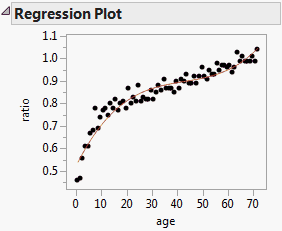Publication date: 11/10/2021

## Polynomial in X to Degree k

Effects to be entered: X, X*X,..., Xk

1. Type k into the text box for Degree.

2. In the Select Columns list, select X.

3. Select Macros > Polynomial to Degree.

Figure 2.6 shows a plot of the data and a cubic polynomial model fit to the data for the Growth.jmp sample data table. This is one of the fits produced when you run the Bivariate data table script.

Figure 2.6 Model Fit for a Degree-Three Polynomial in One Variable